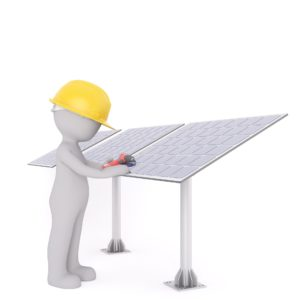# Home Server solar powered, is it feasible? -Part 3

A home server running 24/7 is noticeable on the energy bill. But what if this solar energy is going to run? Is it possible, what are the costs and is it profitable? In this blog I take you along in this research. I'm new to this world (have seen some videos here and there and read things), so maybe I'll make mistakes. Do you see improvement points? I hear them like 😊

Part 2 calculates the amount of capacity required to run the home server 24/7 on solar energy. But, have I made no mistake in this calculation?If unit is reckoned with Watt per hour. But 100 watts operating at 12 volts and 100 watts at 230 volts are not comparable:-(.

Let us bring everything back to the same unit, which should be equal, amperes.

"Watt (W) is the unit of power. Ampere (I) is the unit of amperage. Volt (V) is the unit of the voltage difference. The relationship between these three is: W = V * I So if there is 2 ampere current running at a voltage difference of 220 volts, then the power consumption is 440 watts. This way you can also calculate the amperage if you know the power and the voltage; I = W/V. A 220v stove from 1000w uses 1000/220 = about 4.5 ampere. "

(https://www.startpagina.nl/v/categorieen/elektronica/vraag/605240/bereken-w-watt-a-ampere/)

So the calculation should be:

Zonampanel (2 pieces): 150/12 = 12.5 ampere

Home server: 100/230 = 0.5 ampereBut here too it seems to me something wrong. Between the solar panels/battery and the server, there is an inverter that converts the 12 volts to the 230.

I assume that this inverter has 10% power loss. How much would this device use then. During a previous search trip I have seen inverters of both 12 and 24 volts. For both but make a calculation immediately. And for completeness, you can recalculate the solar panels to 24 volts.

12 volt inverter: 110/12 = 9.2
24 Volt inverter: 120/14 = 5
Solar panels (2 PCs, 24 volts): 150/24 = 6.2

OK, counting the 24 volts, these two panels don't seem to be enough either. In the previous part we went out 8 hours a day and yielded 50%. Per day we are required: 5 A * 24 hours = 120 A. Per day the panels supply: 6.2 A * 0.5 (yields) * 24 = 74 A.

Quite interesting, with this calculation we do not have six, but four panels are needed to achieve the goal.

One thing I totally forgot so far, is that we should also store the stream……

Assuming that the server/inverter uses 2.5 amps per hour and there is daylight for 8 hours, there would be minimal storage required:

120 A (from the previous calculation) – (8 * 2.5) = 100 A. Oops. Let's think about this in the next part………..